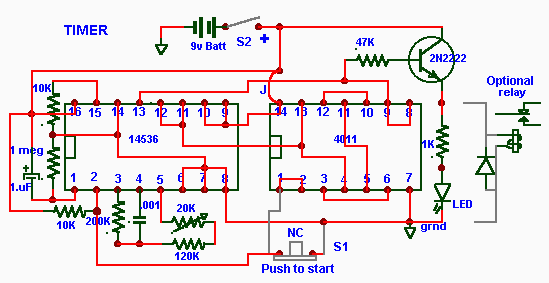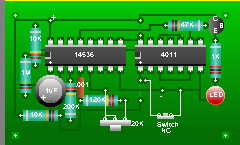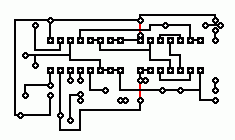#8

While the Timer-Alarm described on Circuit No7 can be calibrated within seconds of the required time, the circuit shown below is the basic timer circuit where the two resistors between pins #4 and #5 R-120k and Variable R-20k are used to calibrate the time delay. Frequency required for each time delay can be calculated for each minute increments i.e... one minute = 140.08khz, while 5 minutes = 140.08khz divided by 5 =28.16khz etc.. A frequency meter is connected at pin #5 of the IC and RV-20k is rotated to obtain the Frequency/time relation and noted by measuring RV-20k resistance or time marked on the enclosure when circuit enclosed.